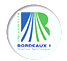Galois Theory and Explicit Methods
Midterm Meeting

Bordeaux, France - October 2008, monday 13th - friday 17thABSTRACTS

 Jean-Marc Couveignes Kummer theory, algebraic groups and fast arithmetic of finite fields (minicourse)
Abstract: There are two standard ways of constructing a degree d finite fields extension FQ/Fq:
We can see FQ as a residue field of the polynomial algebra Fq[x]. More precisely FQ=Fq[X]/f(X) where f(X) is a degree d irreducible polynomial of Fq[X]. In such a model, addition and multiplication can be performed very efficiently but the Frobenius automorphism mapping X to X^q modulo f(X) usually takes a very inelegant form. Another option is to look for a normal basis of FQ/Fq. The main computational advantage of normal basis is that they allow fast exponentiation by q since it corresponds to a cyclic shift of coordinates. There is a concern however about how difficult is multiplication in this context. One can reconciliate these two approaches using Kummer theory of elliptic curves over finite fields. This theory is quite rich because there are many rational torsion points. Let I : E /Fq --> E'/Fq be a quotient by Z/dZ isogeny and let a be a well chosen point in E'(Fq). Assume the fiber B=I^{-1}(a) is irreducible. The residue field Fq(B) is then FQ and automorphisms of FQ/Fq are expressed in terms of translations in E. Using this geometric frame and nice identities between simple elliptic functions, one can construct models for finite fields extensions that cumulate the advantages of either appoaches above.

 Bart de Smit Standard models of finite fields
Abstract:

 Jordi Guárdia Higher Newton polygons in the computation of discriminants and prime ideal decomposition in number fields
Abstract: We present an algorithm for computing discriminants and prime ideal decomposition in number fields, based on Newton polygons of higher order. The running-time and memory requirements of the algorithm appear to be very good: for a given prime number p, it computes the p-valuation of the discriminant and the factorization of p in a number field of degree 1000 in a few seconds in a personal computer.

 Mirjam Jöllenbeck Generalized braid groups, mapping class groups and Galois action
Abstract: After a review of Artin's classical braid groups, we introduce generalized braid groups, also called Artin groups. We present some results of Matsumoto and Paris-Labruere who gave presentations of mapping class groups in terms of Artin groups. Then, we consider their profinite completions and the action of the absolute Galois group G_Q on some examples.

 Bertrand Meyer Minimal heights on the flag variety and designs
Abstract: We introduce the generalised Hermite invariant as the minimum of a twisted height of a flag of vector spaces and the Hermite constant as the maximum of these invariants. As in the classical VoronoÃ¯ theory, we show how to characterise the local maxima of the invariant by two properties known as perfection and eutaxy when the height varies. Then restricting ourselves to the field of rationals, we exhibit examples of extrema related to some root lattices, the Leech lattice or Barnes-Wall lattices by introducing a theory analogous to spherical designs and arguments of group theory.

 Santiago Molina Cerednik-Drinfeld's models of Shimura curves and reduction of CM-points
Abstract:

 Anna Morra Counting cubic extensions with given quadratic resolvent
Abstract: Let k be a number field. Counting extensions of k of fixed degree and bounded discriminant, possibly adding conditions on their Galois closure, is a widely treated problem in number theory. After a quick review of some known results, we will focus on a recent joint work with Henri Cohen. The aim is to count isomorphism classes of cubic extensions K over k whose Galois closure contains a fixed quadratic subextension K_2. We give an explicit asymptotic formula for these classes ordered by the norm of their relative discriminant ideal. The main tool is Kummer theory. The case of cyclic cubic extensions can also be treated, with similar methods.

 Elad Paran Galois theory over complete local domains
Abstract: Complete locals domains play an important role in commutative algebra and algebraic geometry, and their algebraic properties were alreday described by Cohen's structure theorem in 1946. However, the Galois theoretic properties of their quotient fields have only recently began to unfold, where partials results were obtained by D. Harbater, K. Stevenson, F. Pop and the speaker. In this talk we present a new and general result - The absolute Galois group of the quotient field of a complete local domain of dimension exceeding 1 is quasi-free.

 Pierre Parent Serre's uniformity in the split Cartan case
Abstract: We show that, for large enough prime number p, the modular curve X_{split}(p) has no other point with values in Q than CM points and the rational cusp. This gives a partial answer to an old question of J.-P. Serre concerning the uniform surjectivity of Galois representations associated to torsion points on elliptic curves without complex multiplication. (Joint work with Yuri Bilu.)

 Leila Schneps Multiple zeta values, Galois theory and effective methods
Abstract: D'une part, j'expliquerai le lien profond qui unit la théorie des valeurs zeta multiples à la théorie de Galois, lien qui est souvent oublié ou mal compris, tant ces deux domaines semblent indépendants à première vue. D'autre part, j'esquisserai l'utilité des méthodes effectives pour dégager certaines propriétés importantes des MZV.

 William Stein Doing Number Theory with Sage (minicourse)
Abstract: I will talk about computing with elliptic curves, number fields, and modular forms in Sage.

 Till Tantau Visualizing Ideas and Concepts Using TikZ (minicourse)
Abstract:

 Jan Tuitman A generalised sparse effective nullstellensatz
Abstract: